# 5TH ANNIVERSARY AUCTION

### Auction Details

Choose Your Layout >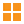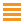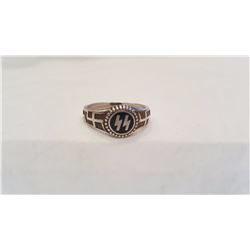2 - Nazi SS Ring
CDC Auctions
Shelf One
Bidding Has Concluded

Sold to f***r for (300.00 + 39.00) x 1 = 339.00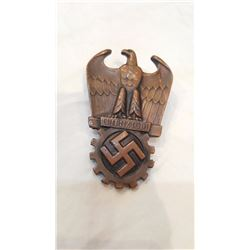3 - Shelf One
CDC Auctions
Shelf One
Bidding Has Concluded

Sold to f***r for (55.00 + 7.15) x 1 = 62.15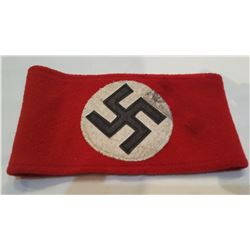4 - Nazi Arm Band
CDC Auctions
Shelf One
Bidding Has Concluded

Sold to f***r for (220.00 + 28.60) x 1 = 248.60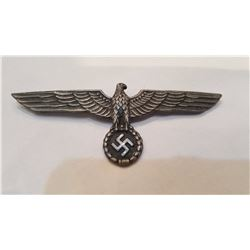6 - Nazi SS Hat Pin
CDC Auctions
Shelf One
Bidding Has Concluded

Sold to f***r for (45.00 + 5.85) x 1 = 50.85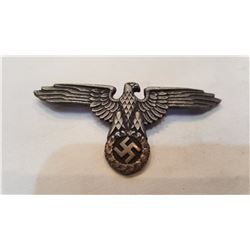23 - Nazi Flight Pin
CDC Auctions
Shelf One
Bidding Has Concluded

Sold to f***r for (75.00 + 9.75) x 1 = 84.75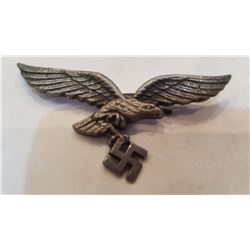25 - Nazi Eagle Pin
CDC Auctions
Shelf One
Bidding Has Concluded

Sold to o****h for (60.00 + 7.80) x 1 = 67.80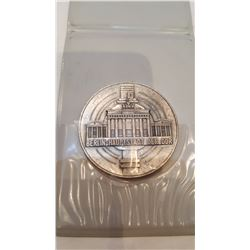27 - WWII Coin And Case
CDC Auctions
Shelf One
Bidding Has Concluded

Sold to f***r for (165.00 + 21.45) x 1 = 186.45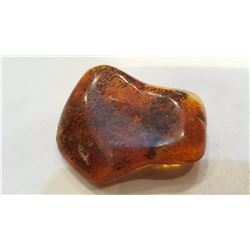28 - Amber Piece
CDC Auctions
Shelf One
Bidding Has Concluded

Sold to f***r for (37.50 + 4.88) x 1 = 42.38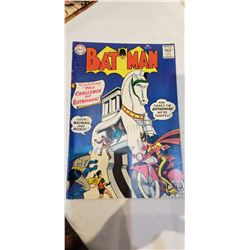40 - Shelf One
CDC Auctions
Shelf One
Bidding Has Concluded

Sold to f***r for (180.00 + 23.40) x 1 = 203.40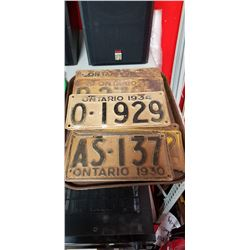43 - Shelf One
CDC Auctions
Shelf One
Bidding Has Concluded

Sold to f***r for (50.00 + 6.50) x 1 = 56.50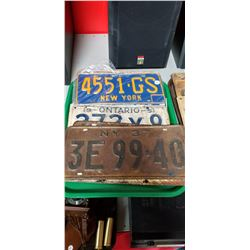44 - Shelf One
CDC Auctions
Shelf One
Bidding Has Concluded

Sold to f***r for (27.50 + 3.58) x 1 = 31.08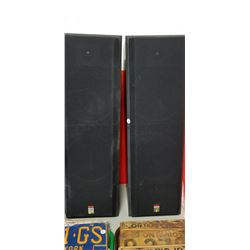45 - Shelf One
CDC Auctions
Shelf One
Bidding Has Concluded

Sold to f***r for (130.00 + 16.90) x 1 = 146.90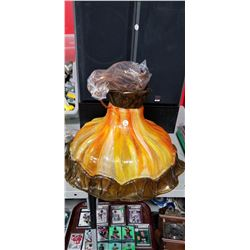46 - Shelf One
CDC Auctions
Shelf One
Bidding Has Concluded

Sold to f***r for (20.00 + 2.60) x 1 = 22.60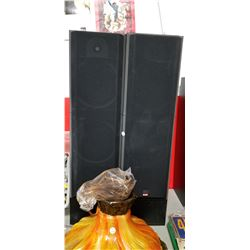47 - Shelf One
CDC Auctions
Shelf One
Bidding Has Concluded

Sold to f***r for (22.50 + 2.93) x 1 = 25.43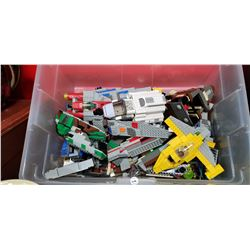49 - Shelf One
CDC Auctions
Shelf One
Bidding Has Concluded

Sold to f***r for (80.00 + 10.40) x 1 = 90.40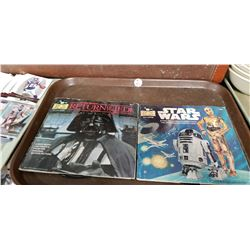50 - Shelf One
CDC Auctions
Shelf One
Bidding Has Concluded

Sold to f***r for (25.00 + 3.25) x 1 = 28.25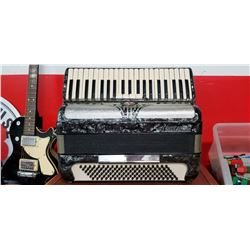51 - Shelf One
CDC Auctions
Shelf One
Bidding Has Concluded

Sold to f***r for (120.00 + 15.60) x 1 = 135.60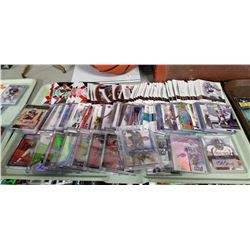52 - Shelf Two
CDC Auctions
Shelf Two
Bidding Has Concluded

Sold to f***r for (22.50 + 2.93) x 1 = 25.43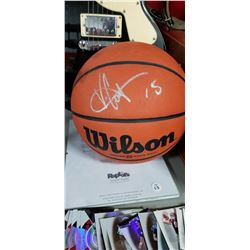53 - Shelf One
CDC Auctions
Shelf One
Bidding Has Concluded

Sold to f***r for (70.00 + 9.10) x 1 = 79.10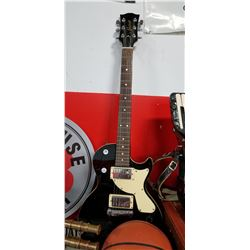54 - Shelf One
CDC Auctions
Shelf One
Bidding Has Concluded

Sold to f***r for (45.00 + 5.85) x 1 = 50.85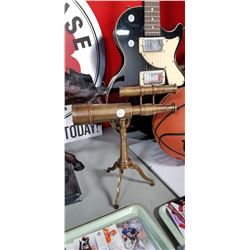55 - Shelf One
CDC Auctions
Shelf One
Bidding Has Concluded

Sold to f***r for (75.00 + 9.75) x 1 = 84.75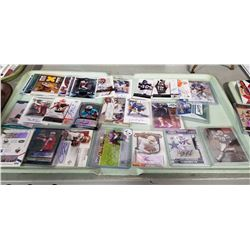56 - Shelf One
CDC Auctions
Shelf One
Bidding Has Concluded

Sold to f***r for (25.00 + 3.25) x 1 = 28.25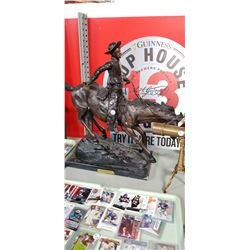57 - Shelf One
CDC Auctions
Shelf One
Bidding Has Concluded

Sold to f***r for (600.00 + 78.00) x 1 = 678.00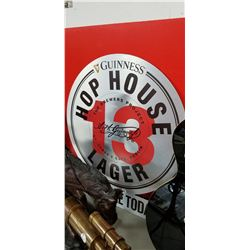58 - Shelf One
CDC Auctions
Shelf One
Bidding Has Concluded

Sold to t*******5 for (15.00 + 1.95) x 1 = 16.95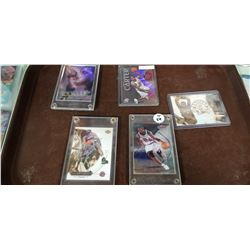59 - Shelf One
CDC Auctions
Shelf One
Bidding Has Concluded

Sold to f***r for (37.50 + 4.88) x 1 = 42.38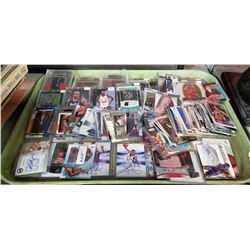60 - Shelf One
CDC Auctions
Shelf One
Bidding Has Concluded

Sold to f***r for (50.00 + 6.50) x 1 = 56.50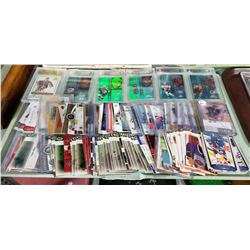61 - Shelf One
CDC Auctions
Shelf One
Bidding Has Concluded

Sold to f***r for (50.00 + 6.50) x 1 = 56.50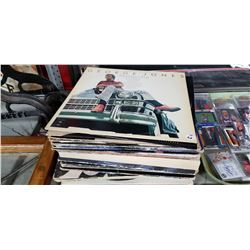62 - Shelf One
CDC Auctions
Shelf One
Bidding Has Concluded

Sold to f***r for (17.50 + 2.28) x 1 = 19.7863 - Shelf One
CDC Auctions
Shelf One
Bidding Has Concluded

Sold to f***r for (475.00 + 61.75) x 1 = 536.75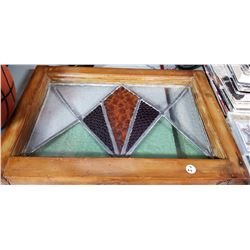64 - Shelf One
CDC Auctions
Shelf One
Bidding Has Concluded

Sold to f***r for (37.50 + 4.88) x 1 = 42.38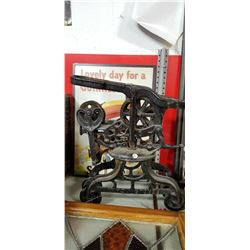65 - Shelf One
CDC Auctions
Shelf One
Bidding Has Concluded

Sold to f***r for (120.00 + 15.60) x 1 = 135.60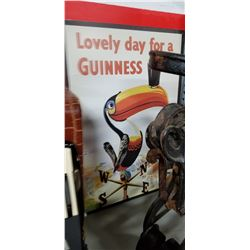66 - Shelf One
CDC Auctions
Shelf One
Bidding Has Concluded

Sold to f***r for (32.50 + 4.23) x 1 = 36.73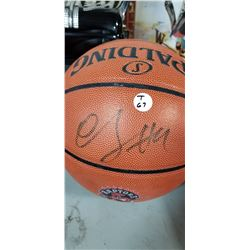67 - Shelf One
CDC Auctions
Shelf One
Bidding Has Concluded

Sold to t*******5 for (75.00 + 9.75) x 1 = 84.75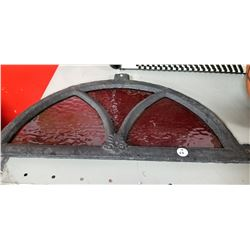68 - Shelf One
CDC Auctions
Shelf One
Bidding Has Concluded

Sold to f***r for (70.00 + 9.10) x 1 = 79.10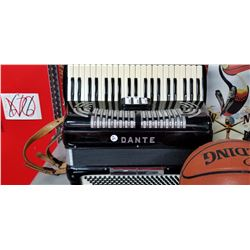69 - Shelf One
CDC Auctions
Shelf One
Bidding Has Concluded

Sold to f***r for (110.00 + 14.30) x 1 = 124.30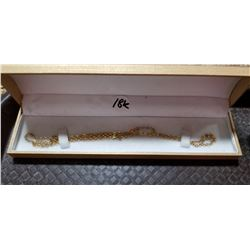73 - Shelf One
CDC Auctions
Shelf One
Bidding Has Concluded

Sold to f***r for (160.00 + 20.80) x 1 = 180.80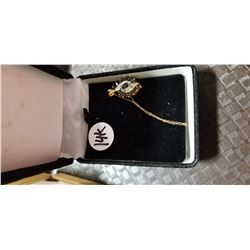74 - Shelf One
CDC Auctions
Shelf One
Bidding Has Concluded

Sold to f***r for (100.00 + 13.00) x 1 = 113.00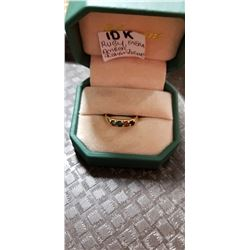75 - Shelf One
CDC Auctions
Shelf One
Bidding Has Concluded

Sold to f***r for (45.00 + 5.85) x 1 = 50.85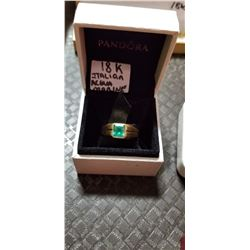76 - Shelf One
CDC Auctions
Shelf One
Bidding Has Concluded

Sold to f***r for (325.00 + 42.25) x 1 = 367.25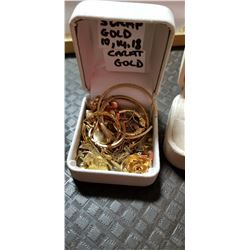77 - Shelf One
CDC Auctions
Shelf One
Bidding Has Concluded

Sold to f***r for (350.00 + 45.50) x 1 = 395.50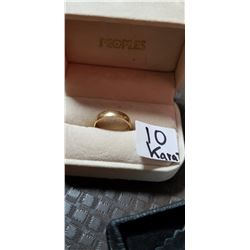78 - Shelf One
CDC Auctions
Shelf One
Bidding Has Concluded

Sold to f***r for (45.00 + 5.85) x 1 = 50.85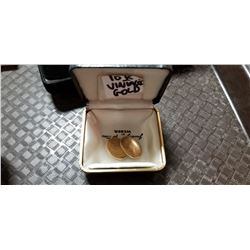79 - Shelf One
CDC Auctions
Shelf One
Bidding Has Concluded

Sold to f***r for (40.00 + 5.20) x 1 = 45.20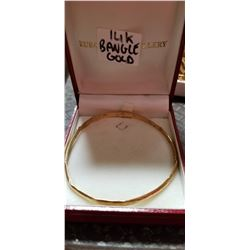80 - Shelf One
CDC Auctions
Shelf One
Bidding Has Concluded

Sold to f***r for (80.00 + 10.40) x 1 = 90.40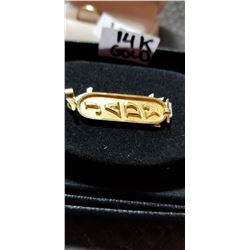81 - Shelf One
CDC Auctions
Shelf One
Bidding Has Concluded

Sold to f***r for (80.00 + 10.40) x 1 = 90.40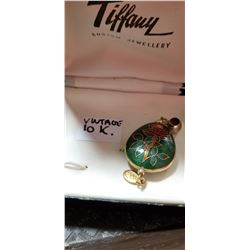82 - Shelf One
CDC Auctions
Shelf One
Bidding Has Concluded

Sold to f***r for (50.00 + 6.50) x 1 = 56.50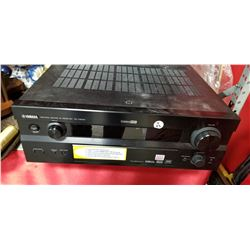83 - Shelf One
CDC Auctions
Shelf One
Bidding Has Concluded

Sold to f***r for (45.00 + 5.85) x 1 = 50.85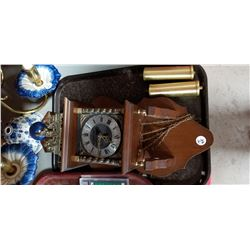85 - Shelf One
CDC Auctions
Shelf One
Bidding Has Concluded

Sold to f***r for (40.00 + 5.20) x 1 = 45.20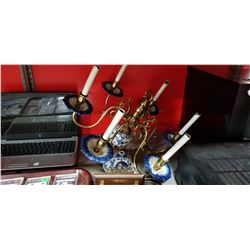86 - Shelf One
CDC Auctions
Shelf One
Bidding Has Concluded

Sold to f***r for (5.00 + 0.65) x 1 = 5.65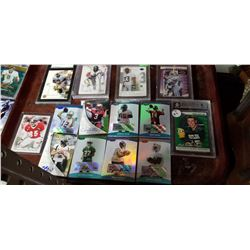87 - Shelf One
CDC Auctions
Shelf One
Bidding Has Concluded

Sold to f***r for (7.50 + 0.98) x 1 = 8.48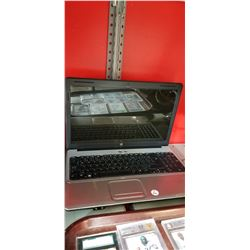88 - Shelf Two
CDC Auctions
Shelf Two
Bidding Has Concluded

Sold to f***r for (60.00 + 7.80) x 1 = 67.80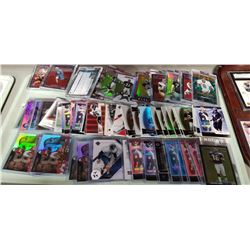89 - Shelf One
CDC Auctions
Shelf One
Bidding Has Concluded

Sold to f***r for (17.50 + 2.28) x 1 = 19.7890 - Shelf One
CDC Auctions
Shelf One
Bidding Has Concluded

Sold to f***r for (70.00 + 9.10) x 1 = 79.10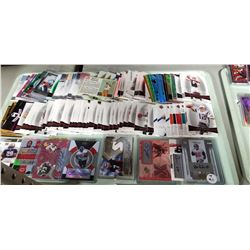91 - Shelf One
CDC Auctions
Shelf One
Bidding Has Concluded

Sold to f***r for (30.00 + 3.90) x 1 = 33.9092 - Shelf One
CDC Auctions
Shelf One
Bidding Has Concluded

Sold to f***r for (40.00 + 5.20) x 1 = 45.2093 - Shelf One
CDC Auctions
Shelf One
Bidding Has Concluded

Sold to f***r for (80.00 + 10.40) x 1 = 90.40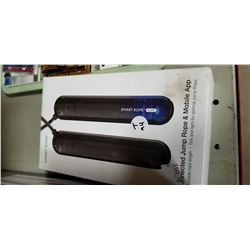94 - Shelf One
CDC Auctions
Shelf One
Bidding Has Concluded

Sold to f***r for (45.00 + 5.85) x 1 = 50.85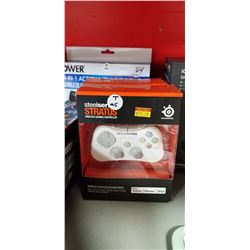95 - Shelf One
CDC Auctions
Shelf One
Bidding Has Concluded

Sold to f***r for (20.00 + 2.60) x 1 = 22.60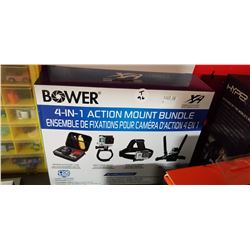96 - Shelf One
CDC Auctions
Shelf One
Bidding Has Concluded

Sold to t*******5 for (20.00 + 2.60) x 1 = 22.60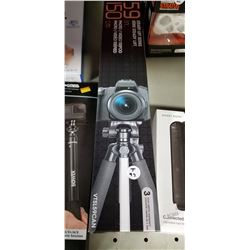97 - Shelf One
CDC Auctions
Shelf One
Bidding Has Concluded

Sold to f***r for (17.50 + 2.28) x 1 = 19.7898 - Shelf One
CDC Auctions
Shelf One
Bidding Has Concluded

Sold to t*******5 for (15.00 + 1.95) x 1 = 16.95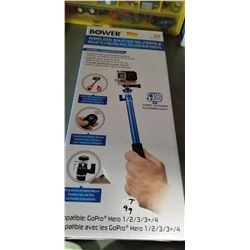99 - Shelf One
CDC Auctions
Shelf One
Bidding Has Concluded

Sold to f***r for (12.50 + 1.63) x 1 = 14.13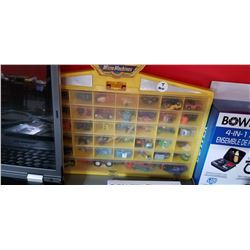100 - Shelf One
CDC Auctions
Shelf One
Bidding Has Concluded

Sold to f***r for (32.50 + 4.23) x 1 = 36.73